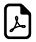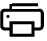Math Eleven Times Tables Tricks11 Times Multiplication Table Trick

An online eleven times table tricks.Simple way to learn 11 times table.Eleven Times Table Simple Learningcan write to
can write to
can write to
can write to
can write to
can write to
can write to
can write to
can write to
can write to
can write to

How 11 times table works?

When you multiply a number by 11, create a blank between two numbers and sum it.

For Example:

Multiply the number 12 by 11, First of all you take the first digit of your number 12 which is 1 and then you imagine a space before you have your second digit which will be the 2.

It should be like 1    ?    2

Now all you want to do is add the both numbers 1 and 2 ,i.e 1 + 2 which gives 3 and you place the 3 in the gap and you get the following.

= 1 3 2

so, answer for 12 x 11 = 132

Think 11 times.Think space between numbers !!!

What math times time do you want to generate ?Times Table Tricks 2 to 11►

2 Times Table Trick

4 Times Table Trick

5 Times Table Trick

6 & 7 Times Table Trick

8 Times Table TrickTop Calculators ►

Tax Calculator, Calories Burned Calculator, PERT, SD Calculator, Dog Age Calculator, Children Equal Playing Time Calculator